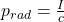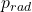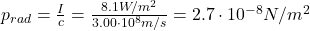## A black, totally absorbing piece of cardboard of area A = 1.7 cm2 intercepts light with an intensity of 8.1 W/m2 from a camera strobe light.

Question

A black, totally absorbing piece of cardboard of area A = 1.7 cm2 intercepts light with an intensity of 8.1 W/m2 from a camera strobe light. What radiation pressure is produced on the cardboard by the light?

in progress 0
2 months 2021-08-02T09:39:29+00:00 2 Answers 1 views 0

2.7×10⁻⁸ N/m²

Explanation:

Since the piece of cardboard absorbs totally the light, the radiation pressure can be found using the following equation:Where:: is the radiation pressure

I: is the intensity of the light = 8.1 W/m²

c: is the speed of light = 3.00×10⁸ m/sTherefore, the radiation pressure that is produced on the cardboard by the light is 2.7×10⁻⁸ N/m².

I hope it helps you!

Explanation:

The expression for total absorption is:

Pr = I/c

Where I = intensity of light

c = velocity of light

Replacing:

Pr = 8.1/3×10⁸ = 2.7×10⁻⁸ Pa